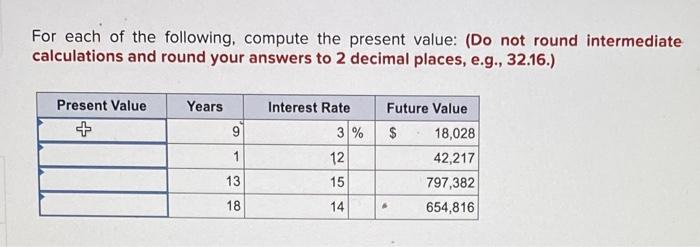# Question For each of the following, compute the present value: (Do not round intermediate calculations and round your answers to 2 decimal places, e.g., 32.16.) For each of the following, compute the present value: (Do not round intermediate calculations and round your answers to 2 decimal places, e.g., 32.16.)